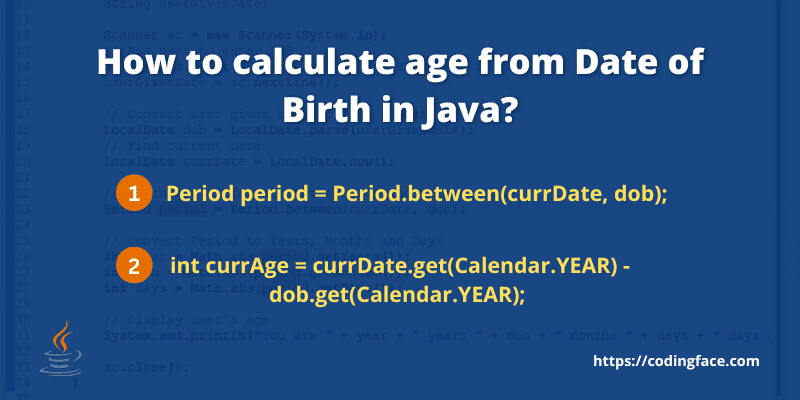# How to calculate age from Date of Birth in Java – 3 easy wayIn this tutorial, We will learn how to calculate age from Date of Birth in Java. We will ask the user to enter their date of birth and from DOB, we will calculate their age also.

## How to calculate age from Date of Birth in Java?

We can calculate age from Date of Birth by using the following methods:

### 1. Calculate age by using Period class

We can calculate the age of the user by using the java.time.Period class.

#### What is java.time.Period class in Java?

The Period class forms a quantity or amount of time in the terms of years, months, and days in the ISO-8601 calendar system such as 26 years, 8 months, and 5 days. Period-supported units are YEARS, MONTHS, and DAYS. These fields are always present and may be set to zero also.

Syntax:

``````public final class Period
extends Object
implements ChronoPeriod, Serializable```Code language: Java (java)```

Let’s see the complete program to calculate age from date of birth by using the Period class.

``````package com.cf.latest;

import java.time.LocalDate;
import java.time.Period;
import java.util.Scanner;

public class CalculateAgeByUsingPeriod {

public static void main(String[] args) throws Exception {
String userGivenDate;

Scanner sc = new Scanner(System.in);

System.out.print("Enter your DOB in YYYY-MM-DD format: ");
userGivenDate = sc.nextLine();

LocalDate dob = LocalDate.parse(userGivenDate);

LocalDate currDate = LocalDate.now();

Period period = Period.between(currDate, dob);

int dobYear = Math.abs(period.getYears());
int dobMon = Math.abs(period.getMonths());
int dobDays = Math.abs(period.getDays());

System.out.print("You age is " + dobYear + " years " + dobMon + " months " + dobDays + " days");

sc.close();
}

}
```Code language: JavaScript (javascript)```

#### Output:

``````Enter your DOB in YYYY-MM-DD format: 1996-12-05
You age is 25 years 9 months 30 days``````

### 2. Calculate age by using Calender class

We can calculate the age of the user by using java.util.Calendar class.

#### What is java.util.Calendar class in Java?

The Calendar class is the abstract class that provides methods for conversion between the specific instant of time and the set of Calendar fields like YEAR, MONTH, DAY_OF_MONTH, HOUR, and more, and for getting customizing calendar fields like getting the date of the next week or last week.

Syntax:

``````public abstract class Calendar
extends Object
implements Serializable, Cloneable, Comparable<Calendar>```Code language: PHP (php)```

Let’s see the complete program to calculate age from date of birth by using the Calendar class.

``````package com.cf.latest;

import java.util.Calendar;
import java.util.GregorianCalendar;

public class CalculateAgeByUsingCalendar {
public static void main(String[] args) {

Calendar calDOB = new GregorianCalendar(1987, 11, 9);

Calendar currDate = new GregorianCalendar();

int currAge = currDate.get(Calendar.YEAR) - calDOB.get(Calendar.YEAR);

if ((calDOB.get(Calendar.MONTH) > currDate.get(Calendar.MONTH))
|| (calDOB.get(Calendar.MONTH) == currDate.get(Calendar.MONTH)
&& calDOB.get(Calendar.DAY_OF_MONTH) > currDate.get(Calendar.DAY_OF_MONTH))) {

currAge--;
}

System.out.println("Your age is " + currAge + " years");
}

}
```Code language: JavaScript (javascript)```

#### Output:

``Your age is 34 years``

## Conclusion

In this tutorial, we have learned how to calculate age from Date of Birth in Java.

We have seen 2 different methods to accept the Date of Birth from the User and calculate the age by using both Period and Calendar classes. You can use any of the above methods to calculate age.

We hope, you can be able to achieve your requirement and like our tutorial. If our tutorial helped you or if you liked our tutorial, then please share it with others.

Thanks & Happy Learning!

## Other Queries We have Covered

• How to accept the date of birth from the user and calculate age in java?
• How to calculate the age of the user in Java?
• What is java.time.Period class in Java?
• What is java.util.Calendar class in Java?

## FAQs

### What is java.time.Period class in Java?

The Period class forms a quantity or amount of time in the terms of years, months, and days in the ISO-8601 calendar system such as 26 years, 8 months, and 5 days. Period-supported units are YEARS, MONTHS, and DAYS. These fields are always present and may be set to zero also.

### What is java.util.Calendar class in Java?

The Calendar class is the abstract class that provides methods for conversion between the specific instant of time and the set of Calendar fields like YEAR, MONTH, DAY_OF_MONTH, HOUR, and more, and for getting customizing calendar fields like getting the date of the next week or last week.

### How to get the current date in Java?

We can get the current date or today’s date by using the Calendar class as below:
Calendar currDate = new GregorianCalendar();